# Problem 53009. Easy Sequences 48: Prime Big Omega of Factorial Sequence

For an integer n, the prime big omega function,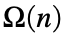, is defined as the total number of prime factors of n. So, if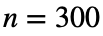, since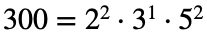, therefore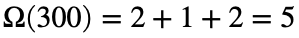.
Given an integer n, write a function that evaluates the following summation:For example for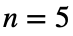:In this case therefore, the function should return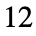.

### Solution Stats

66.67% Correct | 33.33% Incorrect
Last Solution submitted on Mar 19, 2023

### Community Treasure Hunt

Find the treasures in MATLAB Central and discover how the community can help you!

Start Hunting!